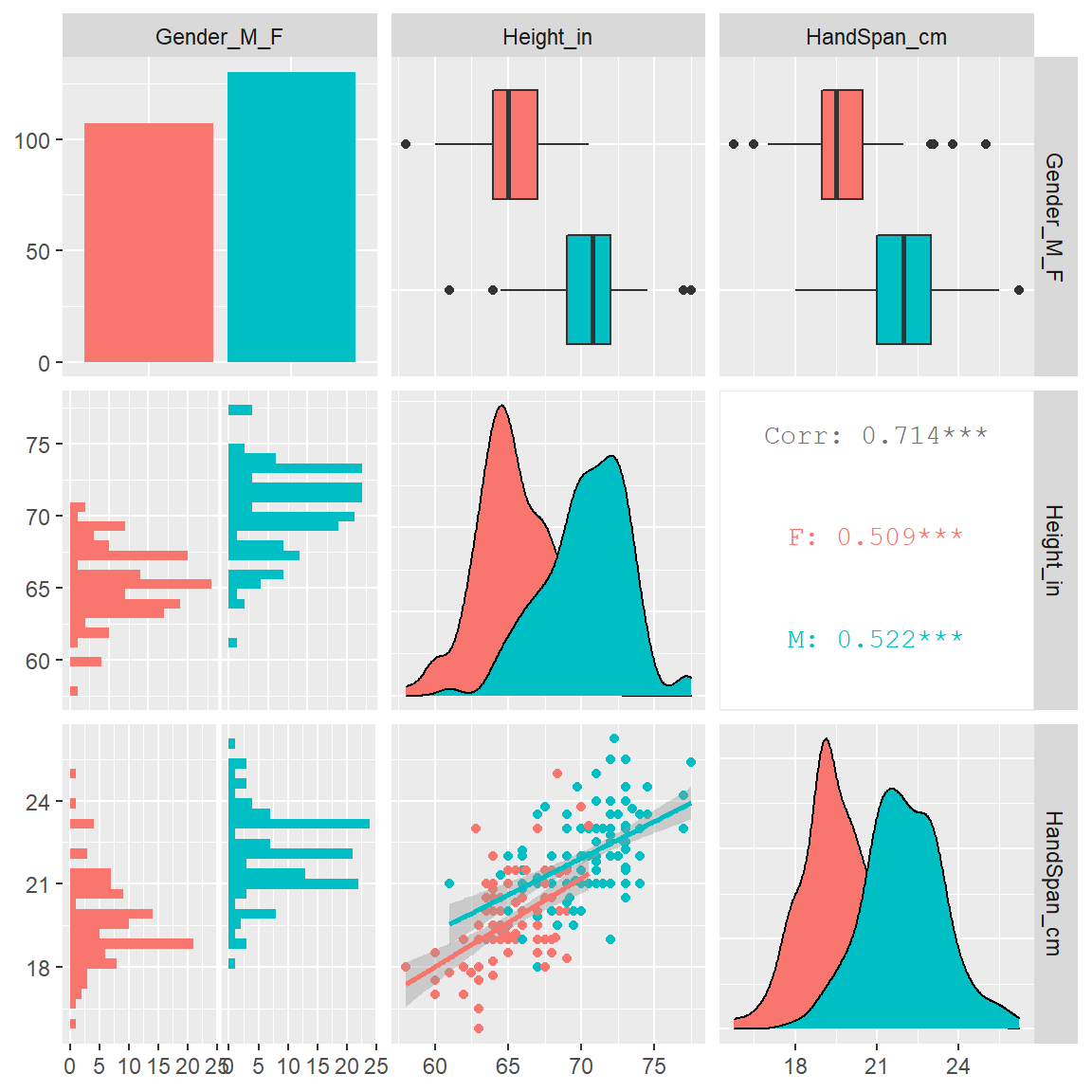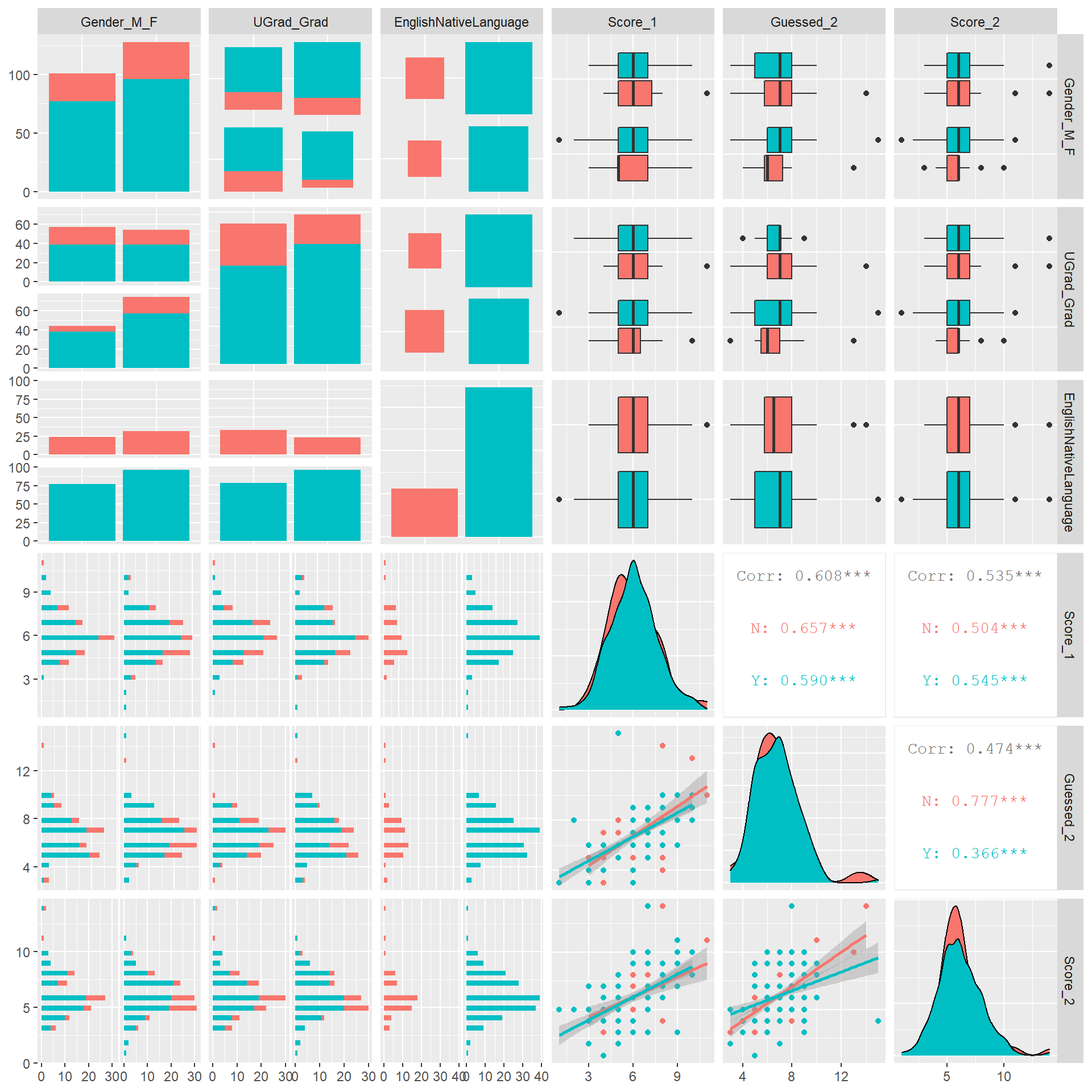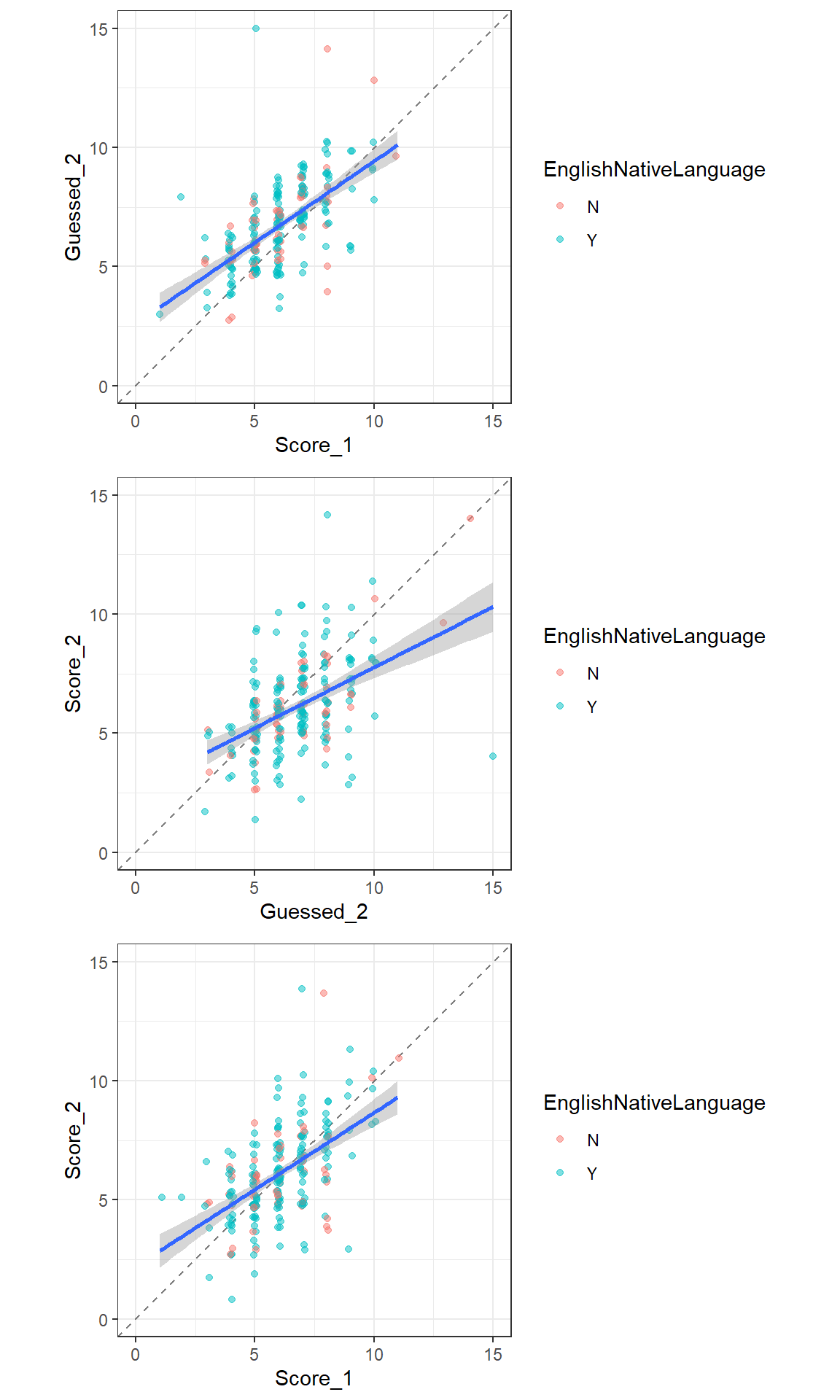Include your answers in this document in the sections below the rubric.

# Rubric

1. (0 p) Participate in two data collection and entering activities.

2. (3 p) Interpret correlation for Males, Females, and Everyone combined.

3. (1 p) How would the correlation change if both hand span and height were measured in inches?

4. (2 p) Why is there a difference in the strength of the correlation for everyone compared to either gender separately?

5. (2 p) Describe the relationships between the scores and the guessed score.

6. (2 p) Identify and explain the most surprising feature of these data.

# Height vs Hand Span

In a previous year, this was the procedure for collecting data:

1. Record your height in inches. For example 5’0" is 60 inches.
2. Use a ruler to measure your hand span in centimeters: the distance from the tip of your thumb to pinky finger with your hand splayed as wide as possible.
4. Analysis.

## Data and Plots

library(tidyverse)
## -- Attaching packages --------------------------------------- tidyverse 1.3.0 --
## v ggplot2 3.3.2     v purrr   0.3.4
## v tibble  3.0.3     v dplyr   1.0.0
## v tidyr   1.1.0     v stringr 1.4.0
## v readr   1.3.1     v forcats 0.5.0
## -- Conflicts ------------------------------------------ tidyverse_conflicts() --
## x dplyr::lag()    masks stats::lag()
# Height vs Hand Span
dat_hand <-
na.omit() %>%
mutate(
Gender_M_F = factor(Gender_M_F, levels = c("F", "M"))
)
## Parsed with column specification:
## cols(
##   Semester = col_character(),
##   Table = col_double(),
##   Person = col_double(),
##   Gender_M_F = col_character(),
##   Height_in = col_double(),
##   HandSpan_cm = col_double()
## )
str(dat_hand)
## tibble [237 x 6] (S3: tbl_df/tbl/data.frame)
##  $Semester : chr [1:237] "F15" "F15" "F15" "F15" ... ##$ Table      : num [1:237] 1 1 1 1 1 1 1 1 2 2 ...
##  $Person : num [1:237] 1 2 3 4 5 6 7 8 1 2 ... ##$ Gender_M_F : Factor w/ 2 levels "F","M": 2 1 1 1 2 2 1 2 2 1 ...
##  $Height_in : num [1:237] 69 66 65 62 67 67 65 70 67 63 ... ##$ HandSpan_cm: num [1:237] 21.5 20 20 18 19.8 23 22 21 21.2 16.5 ...
##  - attr(*, "na.action")= 'omit' Named int [1:141] 9 13 14 15 16 17 18 22 23 24 ...
##   ..- attr(*, "names")= chr [1:141] "9" "13" "14" "15" ...
cor_dat_hand <-
tribble(
~Gender, ~Corr
, "All", dat_hand %>%
summarize(corr = cor(Height_in, HandSpan_cm)) %>% pull()
, "M"  , dat_hand %>% filter(Gender_M_F == "M") %>%
summarize(corr = cor(Height_in, HandSpan_cm)) %>% pull()
, "F"  , dat_hand %>% filter(Gender_M_F == "F") %>%
summarize(corr = cor(Height_in, HandSpan_cm)) %>% pull()
)
cor_dat_hand
## # A tibble: 3 x 2
##   Gender  Corr
##   <chr>  <dbl>
## 1 All    0.714
## 2 M      0.522
## 3 F      0.509
# Plot the data using ggplot and ggpairs
library(ggplot2)
library(GGally)
## Registered S3 method overwritten by 'GGally':
##   method from
##   +.gg   ggplot2
p1 <- ggpairs(dat_hand %>% select(Gender_M_F, Height_in, HandSpan_cm)
, mapping = ggplot2::aes(colour = Gender_M_F)
, lower = list(continuous = "smooth")
, diag  = list(continuous = "density")
#, upper = list(params = list(corSize = 6))
)
## Warning in check_and_set_ggpairs_defaults("diag", diag, continuous = "densityDiag", : Changing diag$continuous from 'density' to ## 'densityDiag' print(p1) ## stat_bin() using bins = 30. Pick better value with binwidth. ## stat_bin() using bins = 30. Pick better value with binwidth.## Questions to answer • Interpret correlation for Males, Females, and Everyone combined. • All: • Male: • Female: • Height was measured in inches and hand span was measured in centimeters. How would the correlation change if both hand span and height were measured in inches? • Why is there a large difference in the strength of the correlation for everyone compared to either gender separately? # Word memory scores 15 seconds to memorize 15 words: http://www.randomlists.com/random-words?qty=15 In a previous year, this was the procedure for collecting data: 1. Round 1 1. Put up a list of words for 15 seconds and view. 2. Have 60 seconds to write/type as many words as you can remember. 3. Score yourself (anonymous, so honesty is best – we’re all going to be bad at this). 2. Given your first performance, make a guess at how many words you’ll remember in round 2. 3. Round 2 (repeat of round 1) 4. Have someone from your table enter your scores at this link (as well as your gender and student status): [link not provided here] 5. Analysis. ## Data and Plots # Memory Scores dat_memory <- read_csv("ADA1_CL_13_Data-CorrMemoryScores.csv") %>% na.omit() %>% mutate( Gender_M_F = factor(Gender_M_F, levels = c("F", "M")) , UGrad_Grad = factor(UGrad_Grad) , EnglishNativeLanguage = factor(EnglishNativeLanguage) ) ## Parsed with column specification: ## cols( ## Semester = col_character(), ## Table = col_double(), ## Person = col_double(), ## Gender_M_F = col_character(), ## UGrad_Grad = col_character(), ## EnglishNativeLanguage = col_character(), ## Score_1 = col_double(), ## Guessed_2 = col_double(), ## Score_2 = col_double() ## ) str(dat_memory) ## tibble [229 x 9] (S3: tbl_df/tbl/data.frame) ##$ Semester             : chr [1:229] "F15" "F15" "F15" "F15" ...
##  $Table : num [1:229] 1 1 1 1 1 1 1 1 2 2 ... ##$ Person               : num [1:229] 1 2 3 4 5 6 7 8 1 2 ...
##  $Gender_M_F : Factor w/ 2 levels "F","M": 2 1 1 1 2 2 1 2 2 1 ... ##$ UGrad_Grad           : Factor w/ 2 levels "G","U": 2 2 2 2 2 2 2 2 2 2 ...
##  $EnglishNativeLanguage: Factor w/ 2 levels "N","Y": 2 2 2 2 2 2 2 1 2 2 ... ##$ Score_1              : num [1:229] 5 8 7 6 8 4 5 8 6 7 ...
##  $Guessed_2 : num [1:229] 5 9 7 5 8 5 6 5 6 6 ... ##$ Score_2              : num [1:229] 6 8 6 5 4 6 4 4 7 7 ...
##  - attr(*, "na.action")= 'omit' Named int [1:149] 9 13 14 15 16 17 18 22 23 24 ...
##   ..- attr(*, "names")= chr [1:149] "9" "13" "14" "15" ...
cor_dat_memory <-
tribble(
~Gender, ~Corr
, "S1-G2", dat_memory %>%
summarize(corr = cor(Score_1, Guessed_2)) %>% pull()
, "G2-S2"  , dat_memory %>% filter(Gender_M_F == "M") %>%
summarize(corr = cor(Guessed_2, Score_2)) %>% pull()
, "S1-S2"  , dat_memory %>% filter(Gender_M_F == "F") %>%
summarize(corr = cor(Score_1, Score_2)) %>% pull()
)
cor_dat_memory
## # A tibble: 3 x 2
##   Gender  Corr
##   <chr>  <dbl>
## 1 S1-G2  0.608
## 2 G2-S2  0.458
## 3 S1-S2  0.569
# Plot the data using ggplot and ggpairs
library(ggplot2)
library(GGally)
, lower = list(continuous = "smooth")
, diag  = list(continuous = "density")
#, upper = list(params = list(corSize = 6))
, progress = FALSE
)
## Warning in check_and_set_ggpairs_defaults("diag", diag, continuous = "densityDiag", : Changing diag\$continuous from 'density' to
## 'densityDiag'
print(p2)
## stat_bin() using bins = 30. Pick better value with binwidth.
## stat_bin() using bins = 30. Pick better value with binwidth.
## stat_bin() using bins = 30. Pick better value with binwidth.
## stat_bin() using bins = 30. Pick better value with binwidth.
## stat_bin() using bins = 30. Pick better value with binwidth.
## stat_bin() using bins = 30. Pick better value with binwidth.
## stat_bin() using bins = 30. Pick better value with binwidth.
## stat_bin() using bins = 30. Pick better value with binwidth.
## stat_bin() using bins = 30. Pick better value with binwidth.library(ggplot2)
p1 <- ggplot(dat_memory, aes(x = Score_1, y = Guessed_2))
p1 <- p1 + theme_bw()
p1 <- p1 + geom_abline(intercept = 0, slope = 1, linetype = "dashed", alpha = 0.5)
p1 <- p1 + geom_jitter(aes(colour = EnglishNativeLanguage), position = position_jitter(width = 0.1), alpha = 1/2)
p1 <- p1 + geom_smooth(method = lm)
p1 <- p1 + scale_y_continuous(limits=c(0, 15))
p1 <- p1 + scale_x_continuous(limits=c(0, 15))
p1 <- p1 + coord_fixed(ratio = 1)
#print(p1)

library(ggplot2)
p2 <- ggplot(dat_memory, aes(x = Guessed_2, y = Score_2))
p2 <- p2 + theme_bw()
p2 <- p2 + geom_abline(intercept = 0, slope = 1, linetype = "dashed", alpha = 0.5)
p2 <- p2 + geom_jitter(aes(colour = EnglishNativeLanguage), position = position_jitter(width = 0.1), alpha = 1/2)
p2 <- p2 + geom_smooth(method = lm)
p2 <- p2 + scale_y_continuous(limits=c(0, 15))
p2 <- p2 + scale_x_continuous(limits=c(0, 15))
p2 <- p2 + coord_fixed(ratio = 1)
#print(p2)

library(ggplot2)
p3 <- ggplot(dat_memory, aes(x = Score_1, y = Score_2))
p3 <- p3 + theme_bw()
p3 <- p3 + geom_abline(intercept = 0, slope = 1, linetype = "dashed", alpha = 0.5)
p3 <- p3 + geom_jitter(aes(colour = EnglishNativeLanguage), position = position_jitter(width = 0.1), alpha = 1/2)
p3 <- p3 + geom_smooth(method = lm)
p3 <- p3 + scale_y_continuous(limits=c(0, 15))
p3 <- p3 + scale_x_continuous(limits=c(0, 15))
p3 <- p3 + coord_fixed(ratio = 1)
#print(p3)

# grid.arrange() is a way to arrange several ggplot objects
library(gridExtra)
##
## Attaching package: 'gridExtra'
## The following object is masked from 'package:dplyr':
##
##     combine
grid.arrange(grobs = list(p1, p2, p3), ncol=1)
## geom_smooth() using formula 'y ~ x'
## geom_smooth() using formula 'y ~ x'
## geom_smooth() using formula 'y ~ x'• Explain the most surprising feature of these data. In particular, think about the Guessed_2 score and how its relationship with the scores differs from between the two scores.